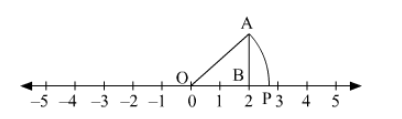# Locate 8–√ on the number line.

Question:

Locate $\sqrt{8}$ on the number line.

Solution:To represent $\sqrt{8}$ on the number line, follow the following steps of construction:

(i) Mark points 0 and 2 as $O$ and $B$, respectively.

(ii) At point $B$, draw $A B \perp O A$ such that $A B=2$ units.

(iii) Join OA.

(iv) With $O$ as centre and radius $O A$, draw an arc intersecting the number line at point $P$.

Thus, point P represents $\sqrt{8}$ on the number line.

Justification:

In right $\triangle \mathrm{OAB}$,

Using Pythagoras theorem,

$\mathrm{OA}=\sqrt{\mathrm{OB}^{2}+\mathrm{AB}^{2}}$

$=\sqrt{2^{2}+2^{2}}$

$=\sqrt{4+4}$

$=\sqrt{8}$